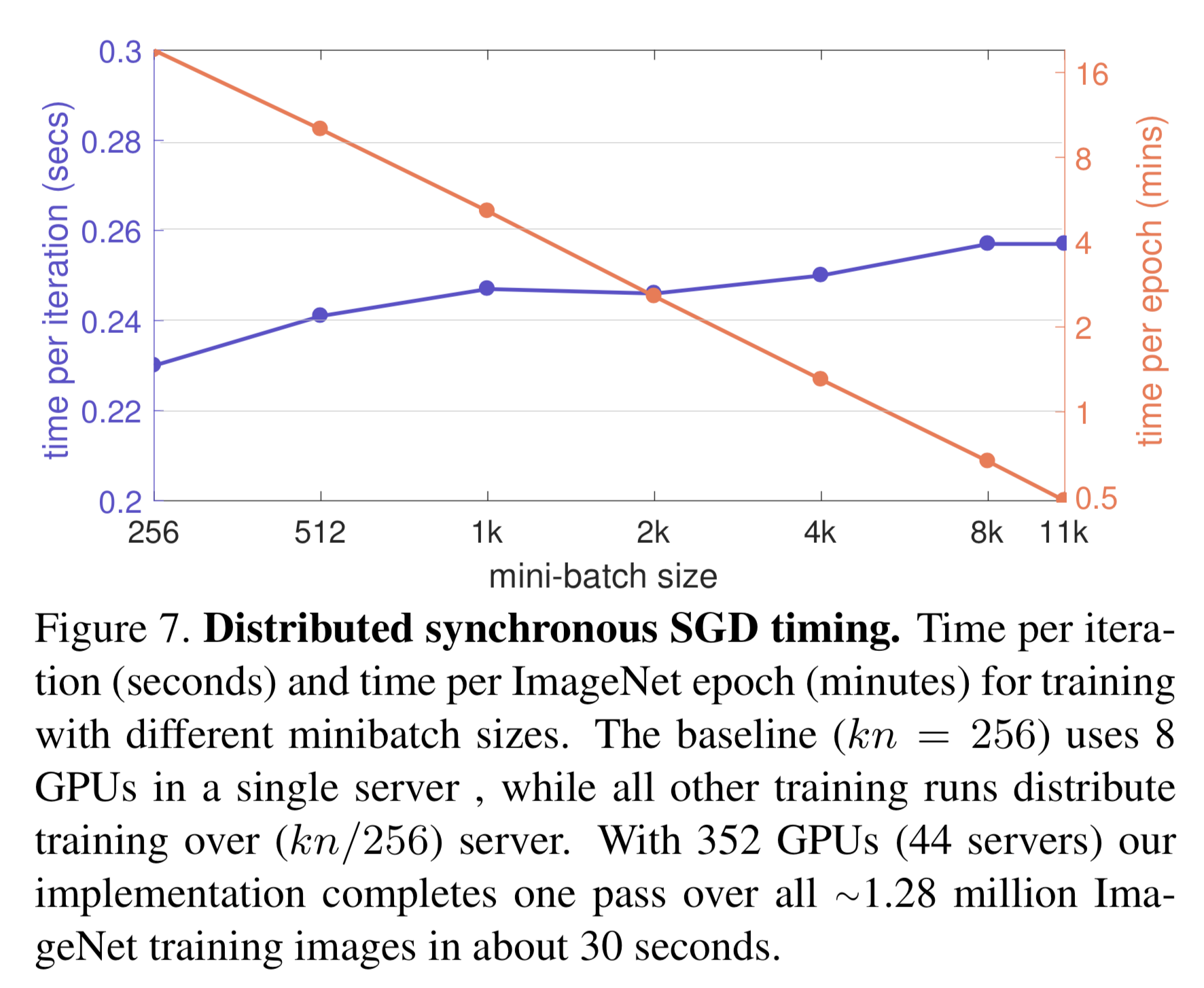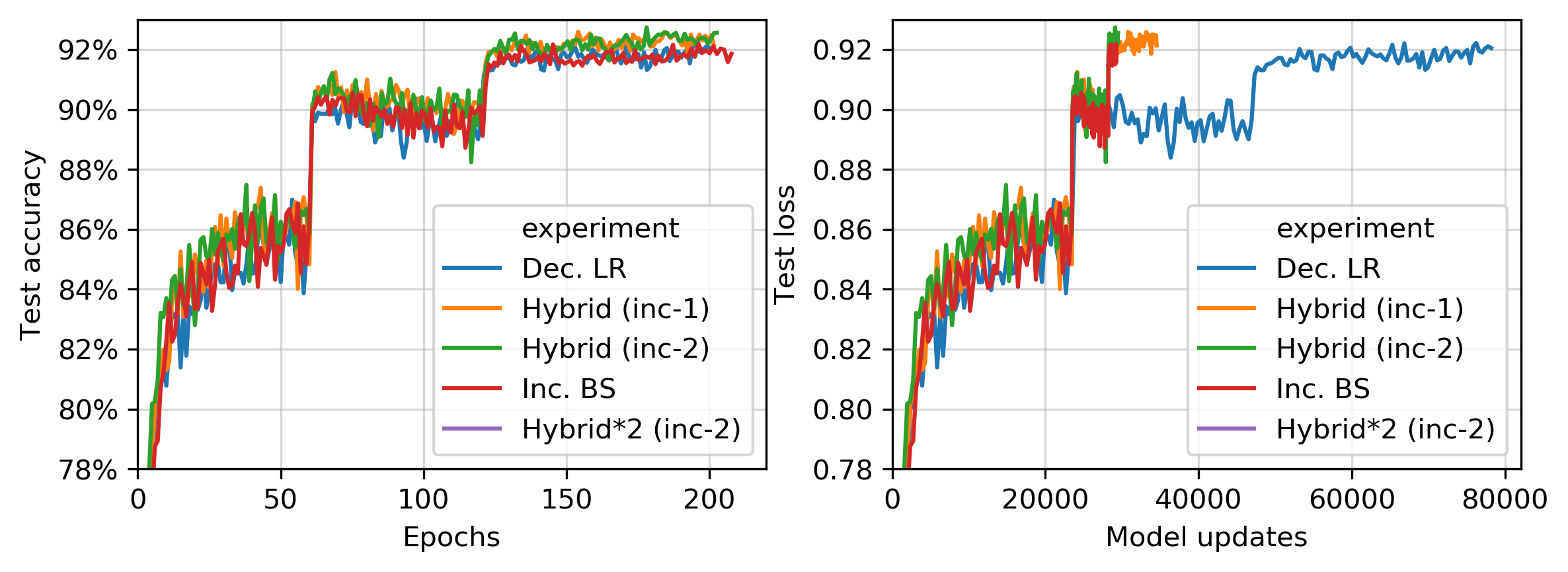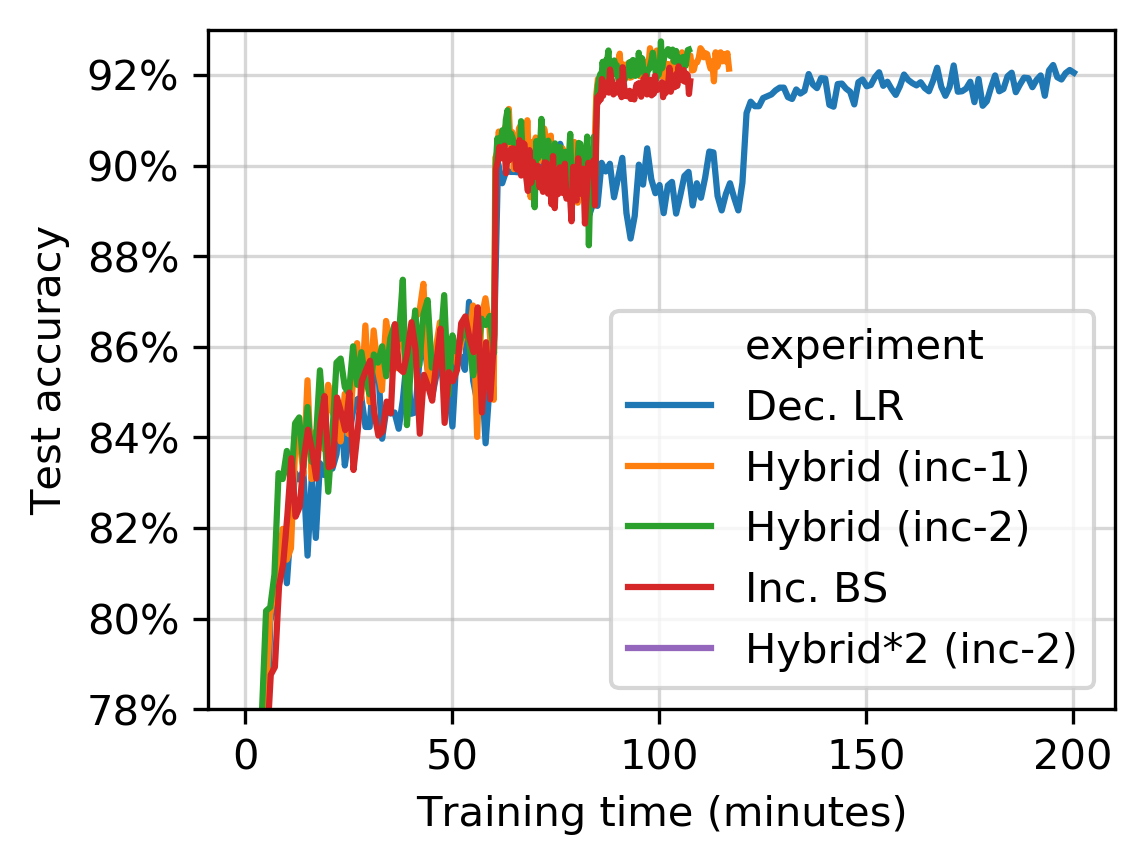# Experiments¶

In Mathematical underpinnings, we showed the method we proposed should require far fewer model updates than SGD while requiring no more floating point operations. The number of model updates can be a good proxy for time spent training, at least with a particular setup of a distributed system [imagenet-1hr].

This page will show experimental results that show using the proposed batch size method results in spending less time training ML models. This will require a particular configuration of a distributed system.

## Synthetic experiments¶

To be updated shortly with experimental results that confirm Mathematical underpinnings. These experiments will be improved versions of the experiments in Section 6.1 of 1.

First, let’s illustrate that the proposed batch size method requires far fewer model updates. To do this, let’s train a neural network (without any activation function) on synthetic data.

$\min_{w_3, W_2, W_1} \sum_{i=1}^{n} (y_i - w_3^T W_2 W_1)^2$

This is a remarkably complicated way to perform linear regression model, but it does introduce some more complexity into the loss function (mostly non-convexity). When we run our adaptive method against other competing methods, we get these results:The loss for various optimizers on the neural network above. Lower values imply a better model. The test loss of the ERM minimizer is shown with the dashed line.

As expected, we find that AdaDamp has a minimal number of model updates and minimal computation:

Of course, the batch size of AdaDamp is intense to compute. However, there are some workarounds to this; the batch size grows closely follows an exponential function as if the bounds were tight. This is some of the motivation behind PadaDamp.

## Distributed system setup¶

Training ML models more quickly requires growing the number of workers with the batch size. That is, if the batch size is 256 there should be 8 workers so each worker computes gradients for 32 examples. If the batch size if 512, there should be 16 workers so the number of gradients computed per worker is constant.

When the distributed is configured this way, a model update takes the same amount time regardless of the batch size [imagenet-1hr]. In their experiments, they increase the batch size by $$44\times$$ and the model update time only takes $$1.12\times$$ longer. That’s shown in their Figure 7:## Distributed experiments¶

Some experiments are (briefly) described below. I am in the process of finishing the simulations on 2021-03-16.

We have mirrored the experiments in Section 5.1 of Smith et al. 2. In these experiments, they present a particular schedule of increasing the batch size that reduces the number of model updates. We have taken their model (a Wide-ResNet model) and dataset (CIFAR10), and followed the same batch size schedule. They run 5 optimizers that only reduce the learning rate or increase the batch size. All other hyperparameters for the optimizer (momentum, weight decay, etc) are the same for every optimizer.

Our software has reproduced their results:The optimizer labeled “Dec. LR” only decreases the learning rate, and the optimizer labeled “Inc. BS” only increases the batch size. The optimziers labeled “Hybrid (inc-$$n$$)” increase the batch size $$n$$ times then start decaying the learning rate afterwards. The experiment labeled “Hybrid*2” has an initial batch size of 256 (instead of 128).

This model has been trained through our software, AdaDamp on NVIDIA T4 GPUs (on an Amazon EC2 g4dn.xlarge instance). When we grow the number of Dask workers with the batch size, these results are produced:This is a simulation. It sets the training time to be the training time on the NVIDIA T4 GPU, and has (very) moderate network bandwidth. We plan to run more simulations that improve the network bandwidth.

1

“Improving the convergence of SGD with adaptive batch sizes”. Scott Sievert and Zachary Charles. 2019. https://arxiv.org/abs/1910.08222

2

“Don’t Decay the Learning Rate, Increase the Batch Size”. Samuel L. Smith, Pieter-Jan Kindermans, Chris Ying, and Quoc V. Le. 2017. https://arxiv.org/abs/1711.00489

imagenet-1hr(1,2)

Section 5.5 of “Accurate, Large Minibatch SGD: Training ImageNet in 1 Hour” P. Goyal, P. Dollár, R. Girshick, P. Noordhuis, L. Wesolowski, A. Kyrola, A. Tulloch Y. Jia, and K. He. 2018. https://arXiv.org/abs/1706.02677